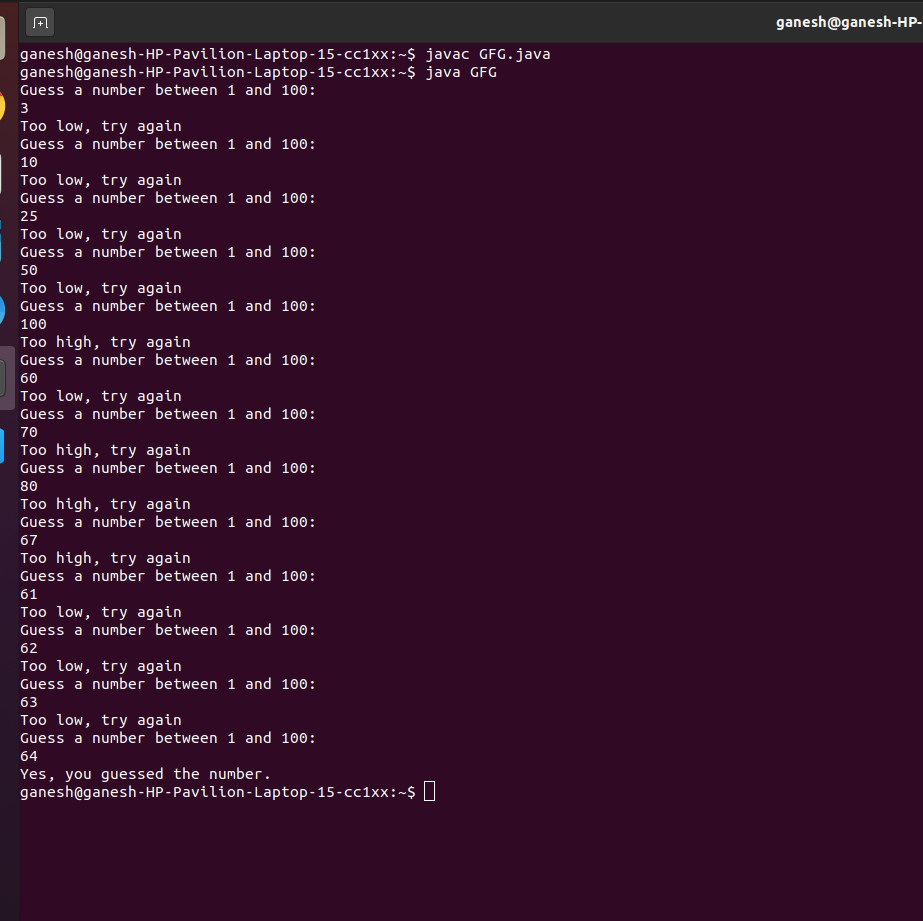Open In App

# Java Program to Guess a Random Number in a Range

Write a program that generates a random number and asks the user to guess what the number is. If the user’s guess is higher than the random number, the program should display Too high, try again. If the user’s guess is lower than the random number, the program should display Too low, try again. The program should use a loop that repeats until the user correctly guesses the random number.

```Input: 15 (a random value that is not known before)
Output: Guess a number between 1 and 100:
1
Too low, try again
Guess a number between 1 and 100:
10
Too low, try again
Guess a number between 1 and 100:
25
Too high, try again
Guess a number between 1 and 100:
20
Too high, try again
Guess a number between 1 and 100:
15
Yes, you guessed the number.```

Example

## Java

 `// Java Program to guess a Random Number Generation`` ` `import` `java.util.Random;``import` `java.util.Scanner;`` ` `public` `class` `GFG {``    ``public` `static` `void` `main(String[] args)``    ``{`` ` `        ``// stores actual and guess number``        ``int` `answer, guess;`` ` `          ``// maximum value is 100``        ``final` `int` `MAX = ``100``;`` ` `        ``// takes input using scanner``        ``Scanner in = ``new` `Scanner(System.in);`` ` `        ``// Random instance``        ``Random rand = ``new` `Random();`` ` `        ``boolean` `correct = ``false``;`` ` `        ``// correct answer``        ``answer = rand.nextInt(MAX) + ``1``;`` ` `        ``// loop until the guess is correct``        ``while` `(!correct) {`` ` `            ``System.out.println(``                ``"Guess a number between 1 and 100: "``);`` ` `            ``// guess value``            ``guess = in.nextInt();`` ` `            ``// if guess is greater than actual``            ``if` `(guess > answer) {``                ``System.out.println(``"Too high, try again"``);``            ``}`` ` `            ``// if guess is less than actual``            ``else` `if` `(guess < answer) {``                ``System.out.println(``"Too low, try again"``);``            ``}`` ` `            ``// guess is equal to actual value``            ``else` `{`` ` `                ``System.out.println(``                    ``"Yes, you guessed the number."``);`` ` `                ``correct = ``true``;``            ``}``        ``}``        ``System.exit(``0``);``    ``}``}`

Output